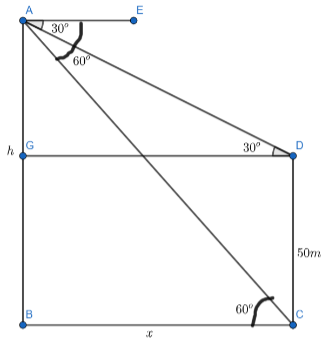Courses
Courses for Kids
Free study material
Free LIVE classes
MoreLIVE
Join Vedantu’s FREE Mastercalss

# The angles of depression of top and bottom of a building $50\,m$ high as observed fromtop of a tower are ${{30}^{o}}$ and ${{60}^{o}}$, respectively. Find the height of the tower and alsothe horizontal distance between the building and the tower.Verified
362.7k+ views
Hint: The given question is related to heights and distance. Try to recall Try to recall the formulae
related to trigonometric ratios and values of trigonometric functions for standard angles.
The following formulae will be used to solve the given problem:
(a) $\tan \theta =\dfrac{opposite\,side}{adjacent\,side}$
(b) $\tan \left( {{30}^{o}} \right)=\dfrac{1}{\sqrt{3}}$
(c) $\tan \left( {{60}^{o}} \right)=\sqrt{3}$

Complete step by step solution:Now, considering the information given in the question, we can draw the following figure for better visualization of the problem:

Let $AB$ be the tower of height $h\,$meter and $CD$ be the building of height $50\,m$ . Let $x$
meter be the horizontal distance between the building and the tower. We will consider a point $G$
on the tower $AB$ which is at the same level as the top of the building $CD$ . So, $BG=50\,m$ and
$AG=\left( h-50 \right)\,m$ . Also, $GD=x$ meter . In the question, it is given that the angle of
depression of top and bottom of the building as seen from the top of the tower are ${{30}^{o}}$ and
${{60}^{o}}$ respectively. So, , $\measuredangle EAC={{60}^{o}}$ and $\measuredangle EAD={{30}^{o}}$.
Now, $AE$ and $BC$ are horizontal lines. So, they are parallel to each other. So, $\measuredangle EAC$ and $\measuredangle ACB$ are alternate interior angles. So, they will be equal. Also,
$\measuredangle EAD$ and $\measuredangle ADG$ are also alternate interior angles. So, they will
also be equal. So, $\measuredangle ACB=\measuredangle EAC={{60}^{o}}$ and $\measuredangle ADG=\measuredangle EAD={{30}^{o}}$.
Now, we will consider $\Delta ADG$.
In $\Delta ADG$ ,
$\tan \left( {{30}^{0}} \right)=\dfrac{h-50}{x}$
$\Rightarrow \dfrac{1}{\sqrt{3}}=\dfrac{h-50}{x}$
$\Rightarrow x=\sqrt{3}\left( h-50 \right)......(i)$
Now, we will consider $\Delta ACB$ .
In $\Delta ACB$,
$\tan \left( {{60}^{o}} \right)=\dfrac{h}{x}$
$\Rightarrow \sqrt{3}=\dfrac{h}{x}$
$\Rightarrow x=\dfrac{h}{\sqrt{3}}......(ii)$
Now, the distance between the tower and the building is the same in both cases. So, $(i)=(ii)$ .
$\Rightarrow \sqrt{3}\left( h-50 \right)=\dfrac{h}{\sqrt{3}}$

On cross-multiplying, we get:
$3\left( h-50 \right)=h$
$\Rightarrow 3h-150=h$
$\Rightarrow 2h=150$
$\Rightarrow h=75\,m$
Substituting $h=75$ in equation $(ii)$ , we get:
$x=\dfrac{75}{\sqrt{3}}\,m$
Hence, the height of the tower is $75\,m$ and the distance between the building and tower is equal
to $\dfrac{75}{\sqrt{3}}\,m$.

Note: Students are generally confused between the values of $\tan \left( {{30}^{o}} \right)$ and $\tan \left( {{60}^{o}} \right)$. $\tan \left( {{30}^{o}} \right)=\dfrac{1}{\sqrt{3}}$ and $\tan \left({{60}^{o}} \right)=\sqrt{3}$. These values should be remembered as they are used in various problems of heights and distances.
Last updated date: 02nd Oct 2023
Total views: 362.7k
Views today: 4.62k